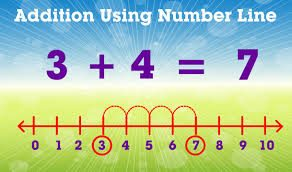# ADDITION, WORD PROBLEMS AND A NUMBER-LINE-P.1

##### This unit involves adding numbers less than 20 both horizontally and vertically,Word problems involving addition and adding numbers on a number line

Adding numbers less than 20 both horizontally and vertically.

A 1) 2 + 7 = 2) 9 + 6 = 3) 13 + 6 = 4) 1 + 4 + 5 = 5) 3 + 3 + 2 =

B 1) 8  2)3) 1 2 4) 2 5)6) 1 6

+1             + 5         +     4                 2               4                + 2

+ 2             + 3

Word problems involving addition

– Reading, interpreting, adding, writing, forming word problems.

e.g. a) 1 house + 2 houses = _______ houses

a)Mummy has 5 bananas. Daddy has 3 bananas.

How many bananas do they have altogether?

= 5 + 3 = ________

They have _______ bananas altogether.

Adding numbers less than 20 on a number line

Drawing a number line

Adding numbers on a number line

e.g. 6 + 3 = ___9_

1) 3 + 6 = 2) 2 + 7 = 3) 9 + 1 = 4) 4 + 5 = 5) 5 + 2 = 6) 3 + 2 =

SEE ALL
•YOU